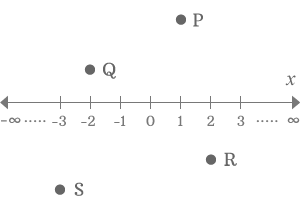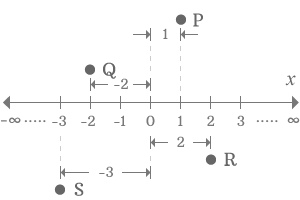# x-axis

A number line which uses to know the exact location of a point on a plane in horizontal direction is called $x$-axis.x-axis is a coordinate axis in Cartesian coordinate system and it is displayed parallel to the surface of the earth. Therefore, it is also called as horizontal axis. It is mainly used to know the horizontal distance of a point on a plane by comparing the horizontal distance of the point with the lengths on the number line.

## Parts

The horizontal x-axis is having three important sections and they are used to identity the exact location of the point on the plane.

1.

### Origin

Origin of x-axis is the exact middle point of the x-axis and it bisects the plane into two equal parts vertically and it is denoted by zero.

2.

### Positive Region

The right side of x-axis is equally divided into numerous equal parts and each division is denoted by a positive real number.

3.

### Negative Region

The left side of x-axis is also equally divided into numerous equal parts and each division is denoted by a negative real number.

#### How to use$P,Q,R$ and $S$ are four points on a plane and they are at four different locations on the plane.

The position of point with respect to x-axis is the horizontal distance of the point. The horizontal distance of the point is measured from the origin of x-axis.

The point $P$ is above the x-axis and also in right side of the origin. So, it is in positive region. The position of point $P$ with respect to x-axis is horizontal distance from the origin. The point $P$ is exactly at the division of $1$. So, the horizontal distance of the point $P$ is $1$ and the location of point $P$ is also $1$.The point $Q$ is also above the x-axis and it is at division $–2$. So, the horizontal distance and the location of point $Q$ on the plane is $–2$.

The point $R$ is below the x-axis but is in right side of origin. The point $R$ is at division $2$. So, the location of point $R$ is $2$.

The point $S$ is below the x-axis but is in left side of the origin. The point $S$ is at division $–3$. So, the position of point is $–3$.

Though the points $P$ and $R$ are above and below the x-axis respectively, their positions are denoted by the positive numbers because they both are right side to the origin of x-axis. So, the points can be above or below the x-axis and the horizontal distance is considered as the position of them. The points $Q$ and $S$ are also above and below the x-axis but they are in left side of x-axis. Therefore, they are denoted by negative numbers.

Latest Math Topics
Latest Math Problems
Email subscription
Math Doubts is a best place to learn mathematics and from basics to advanced scientific level for students, teachers and researchers. Know more
Follow us on Social Media
###### Math Problems

Learn how to solve easy to difficult mathematics problems of all topics in various methods with step by step process and also maths questions for practising.

Learn more